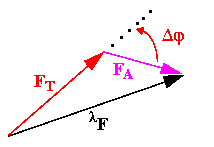# MAD phasing - part 1

### Basic vector relationships

The diagram below illustrates the vector relationships among three quantities that we will estimate for each Bragg reflection h by measuring the scattering amplitude Fobs() for several wavelengthsi.

### Phase diagram for MAD phasing= total observed scattering amplitude from our diffraction measurement at wavelength, which has an unknown phase we would dearly like to know
FT = Normal scattering component of all atoms
FA = Anomalous scattering contribution from all atoms= Difference in phase angle between normal and anomalous scattering components

The basic idea is that if we can locate the anomalous scattering atoms within the unit cell (those contributing to FA) then we can calculate the corresponding phase angleA. The MAD phasing equations of Karle  (which we will get to in a minute) can then be used to generate an estimate forand FT. In the simplest case (we will later show more sophisticated estimates) we can then estimate the phase of the FT as+A. A Fourier transform of the amplitudes FT and phases (+A) should yield an electron density map corresponding to all atoms in the structure.

We may further break down the anomalous scattering component into contributions from several types of anomalous scatterers. Each anomalous scattering type will have its own wavelength-dependent scattering behaviour, which we will write:back to indexnext topic
Ethan A Merritt ©1995-2001/ merritt@u.washington.edu / Biomolecular Structure Center at UW